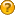###Author Topic: project3D2D: how to tell if center is behind the viewplane and clip  (Read 2880 times)

####stormp##### project3D2D: how to tell if center is behind the viewplane and clip
« on: August 13, 2007, 09:35:39 pm »
Hi,

Still working on my grid lines.project3D2D wont render if "the center is behind the viewplane".   How can I adjust my vectors to clip at the approriate end?

Much thanks.

S.

Code: [Select]
`        private void drawLine(FrameBuffer fb, Graphics2D g, SimpleVector v1, SimpleVector v2)    {        SimpleVector point1 = Interact2D.project3D2D(theWorld.getCamera(), fb, v1);        SimpleVector point2 = Interact2D.project3D2D(theWorld.getCamera(), fb, v2);        if(point1 != null && point2 != null)        {            g.drawLine((int)point1.x, (int)point1.y, (int)point2.x, (int)point2.y);        }  // if    } `

####EgonOlsen

••` SimpleVector start=new SimpleVector(10,10,10); SimpleVector end=new SimpleVector(30,30,-10); float planeAt=1.01f; SimpleVector dir=end.calcSub(start); float mul=(planeAt-start.z)/dir.z; float x=start.x+mul*dir.x; float y=start.y+mul*dir.y; SimpleVector intersect=new SimpleVector(x,y,planeAt);`